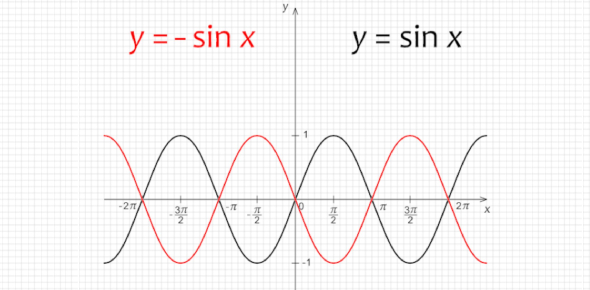# Trigonometric Graphs Test

10 Questions | Total Attempts: 176SettingsCreate your own QuizHow good is your trigonometric knowledge? Take this trigonometric graphs test, and see how well you practiced trigonometry functions and graphs. If you have been practicing the topic well, it will be an easy quiz for you. Give it a try, and check out your scores. We have a set of questions where you have to study the trigonometry graph and accordingly give the answer. All the best! Do share the quiz with others and help them practice trigonometric graphs.

• 1.
What is the shape of a cosine graph like?
• A.

Banana

• B.

Dracula Teeth

• C.

Mountain

• D.

Orange

• 2.
What is the amplitude of the graph y = 2sin(x+pi/4)
• 3.
What is the period of the graph y = tan 5x. Answer in degrees.  You do not have to write the word "degrees."
• 4.
What is the amplitude of the graph y = tan 2x?
• 5.
What is the period of the graph y = tan 3x?Answer in degrees You do not have to write the word "degrees"
• 6.
Sketch the graph of y = 2 sinx -1 and state its rangeLeave your answer as "a
• 7.
Sketch the graph of y = 3 - cos2x and state its range. Leave your answer as "a
• 8.
Sketch the graphs y=4sin 2x and y = 2cosx -1 for 0<360 degrees on the same diagram.How many solutions are there in this interval for 4 sin2x + 1 = 2 cosx?
• 9.
Sketch the graphs of y = 2|cos2x| and y = 1+sinx for 0<360 degrees.What is the amplitude of the graph y =1+sinx?
• 10.
From the previous question, find the number of distinct values of x in this interval for which: 2cos2x = sinx+1
Related TopicsBack to top
×

Wait!
Here's an interesting quiz for you.